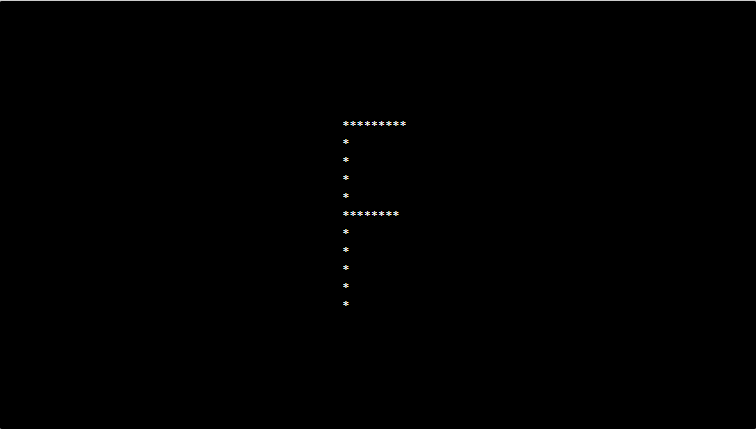# PHP program to print alphabet pattern F

In this article write a PHP program to print alphabet pattern F. This Program first takes the numbers of rows and then prints pattern using nested for loops.

## PHP program to print alphabet pattern F

```<?php
echo "<pre>";
for (\$row = 0; \$row < 11; \$row++) {
for (\$col = 0; \$col <= 11; \$col++) {
if (\$col == 1 or (\$row == 0 and \$col > 1 and \$col < 10) or (\$row == 5 and \$col > 1 and \$col < 9)) {
echo "*";
} else {
echo "&nbsp;";
}
}
echo "<br/>";
}
echo "</pre>";
?>
```

### Output: